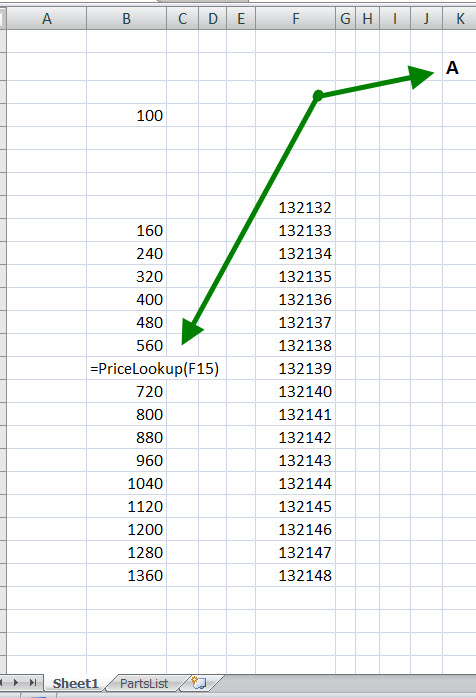# User-Defined Functions

## Lookup Function

I received an email from Clark regarding a formula about a foot long including a VLOOKUP and 3 nested IFs.

Fixing the basic formula was easy; then I suggested a Custom Function to replace typing that whole thing. This is the result.

```Public Function PriceLookup(PartNum As Variant)

' +-----------------------------------------------------------------------********---------+
' |   The original formula
' |    =VLOOKUP(F8,PartsList!\$D\$2:\$K\$9899,IF(K2="A",5,IF(K2="B",6,IF(K2=C,7,0))),FALSE)
' +----------------------------------------------------------------------------------------+
Dim ColLook     As Long
Select Case Range("K2")     'Look for letter here
Case "A", "a"
ColLook = 5
Case "B", "b"
ColLook = 6
Case "C", "c"
ColLook = 7
Case Else
ColLook = 10        'Basically throw error

End Select
PriceLookup = Application.VLookup(PartNum, [PriceList], ColLook, False)
'VLOOKUP(theCell, Named_Lookup_Range, Which_Column,Exact_Match)
End Function
```

See the illustration below for how the function is used.